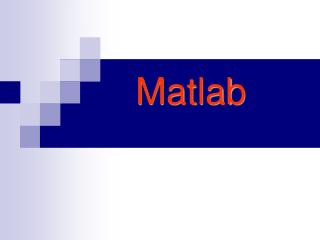DownloadDownload PresentationMatlab

# Matlab

Download Presentation## Matlab

- - - - - - - - - - - - - - - - - - - - - - - - - - - E N D - - - - - - - - - - - - - - - - - - - - - - - - - - -
##### Presentation Transcript

1. Matlab

2. 利用MATLAB进行信号与系统分析 • MATLAB简介 • 信号的MATLAB表示 • 利用MATLAB进行系统的时域分析 • 利用MATLAB进行信号的频域分析 • 利用MATLAB分析系统的频率特性 • 利用MATLAB进行连续系统的s域分析 • 利用MATLAB进行离散系统的z域分析 • 利用MATLAB进行系统的状态变量分析

3. MATLAB简介 ( Matrix Laboratory ) • MATLAB的工作方式 • 如何获取帮助 • 表达式——变量、数值、算数运算符、关系运算符、逻辑运算符、冒号运算符 • 数组及其运算 • 函数文件 • 循环（FOR、 WHILE循环） • 基本绘图语句

4. 一、MATLAB的工作方式 （1）窗口命令方式 （2）运行以 .M为扩展名磁盘文件

5. 工作方式举例 %用plot函数画一个方波 t=[-1 0 0 1 1 3]; x=[0 0 1 1 0 0]; plot(t,x); xlabel('t');ylabel('x(t)'); axis([-1 3 0 2]); 直接在命令窗口输入以上命令 建一个名字为my_file.M的文件，然后在命令窗口输入文件名及回车。

6. 二、获取帮助 命令窗口输入： help+函数名 例如 help plot

7. 三、表达式 • 变量 • 不需要变量的类型说明 • 变量名的第一个字符必须是字母 • 变量名长度：不超过31个字符 • 大写和小写的字母视为不同的字符 例如：num_students = 25 • 特殊变量： pi 表示圆周率，inf表示无穷大，NaN(Not a Number)表示不定量，如0/0。

8. 三、表达式 • 数值 • MATLAB用常规的十进制表示数值 • 用i或j作为后缀来表示复数的虚部 例 1.235e5表示1.235105，x=2+3j abs(x)求复数x的模 angle(x)求复数x的相角(弧度) real(x)求复数x的实部 imag(x)求复数x的虚部 conj(x)求复数x的共轭

9. 三、表达式 • 运算符号 • 算数运算符 • +加 • -减 • * 乘 • / 除 • ^ 乘方 • ‘ 矩阵的复共轭转置

10. 三、表达式 • 运算符号 • 逻辑运算符 • A & B 逻辑与(and) • A | B 逻辑或(or) • ~A 逻辑非(not) 值为0时表示逻辑假(F)，其它任何非零值表示逻辑真。

11. 三、表达式 • 运算符号 • 关系运算符 • A < B 小于 • A > B 大于 • A <= B 小于等于 • A >= B 大于等于 • A == B 等于 • A ~= B 不等于

12. 三、表达式 • 运算符号 • 冒号运算符 • 表达式 1:10表示产生一个行向量，它的值为 1 2 3 4 5 6 7 8 9 10 • 表达式 10:-2:1表示产生一个递减的行向量，它的值为 10 8 6 4 2

13. 四、数组 1. 数组的构造 用冒号:产生数组 例 x=2:5产生一个数组，它的值为 x(1)=2, x(2)=3, x(3)=4, x(4)=5 用linspace产生数组 x=linspace(0,2,11)将区间[0，2]均匀抽样11点作为数组x 给2维数组赋值时，用分号表示一行的结束，如：z=[1 2; 3 4]。

14. 四、数组 1. 数组的构造 MATLAB 提供了一些产生基本矩阵的函数 zeros产生矩阵元素全为0的矩阵 ones产生矩阵元素全为1的矩阵 rand产生(0,1)均匀分布随机数矩阵 randn产生正态分布随机数矩阵

15. 四、数组 2. 数组的运算 • 数组和一个标量相加或相乘 例 y=x-1 z=3*x • 2个数组的对应元素相乘除 .* ./ 例 z=x.*y • 确定数组大小的函数 size(A)返回值数组A的行数和列数（二维） length(B)确定数组B的元素个数（一维）

16. 五、函数文件 • M文件的第一行包含function • 功能: 建立一个函数，可以同MATLAB的库函数一样使用。

17. 五、函数文件 例：编一个绘制图示波形的函数。 function y=tri(t) y=[ abs(t)<=1].*(1-abs(t)); 调用函数tri，并画出它的波形 t=-2:0.05:2; plot(t,tri(t));

18. 六、For 循环 例:编写计算s=1+2+3+…+100的MATLAB程序 s=0; for n=1:100 s=s+n; end

19. 七、While 循环 例:计算 的值，且误差小于10-6 s=0; n=1; eps=1e-6; while 1/(n*n) > eps s=s+1/(n*n); n=n+1; end fprintf('s=%.5f\n',s)

20. 八、plot函数——绘图函数(continuous) t=linspace(0,4*pi,512); plot(t,sin(t),t,cos(t),'-.'); title('my figure'); xlabel('t'); legend('sin(t)','cos(t)');

21. 八、plot函数——绘图函数(continuous)

22. 九、stem函数——绘图函数(discrete) k=0:39; stem(k,cos(0.9*pi*k)); title('cos(0.9\pik)');

23. 九、stem函数——绘图函数(discrete) cos(0.9k)波形

24. 信号的MATLAB表示 • 基本信号的MATLAB表示 指数信号Aeat 、指数序列ak 、抽样函数Sa(t)、 正弦型信号、矩形脉冲信号、三角脉冲信号 • 信号基本运算的MATLAB实现 尺度变换、翻转、时移、 相加、相乘、 差分与求和、微分与积分

25. 一、基本信号的MATLAB表示 • 指数信号Aeat y = A*exp(a*t); • 指数序列ak 幂运算a.^k实现 • 正弦型信号 内部函数cos( ) 和sin( ) • 抽样函数Sa(t) sinc(t) • 矩形脉冲信号 y = rectpuls(t,width) • 三角波脉冲信号 y = tripuls(t, width,skew)

26. 一、基本信号的MATLAB表示 %decaying exponential t=0:001:10; A=1; a=-0.4; ft=A*exp(a*t); plot(t,ft) t=0:0.1:10; A=1; a=-0.4; ft=A*exp(a*t); stem(t,ft)

27. 一、基本信号的MATLAB表示 % rectpuls t=0:0.001:4; T=1; ft=rectpuls(t-2*T,T); plot(t,ft) axis([0,4,-0.5,1.5])

28. 一、基本信号的MATLAB表示 % tripuls t=-3:0.001:3; ft=tripuls(t,4,0.5); plot(t,ft) ft=tripuls(t,4,1);

29. 一、基本信号的MATLAB表示 % unit impuls sequence k=-50:50; delta=[zeros(1,50),1,zeros(1,50)]; stem(k,delta) function [f,k]=impseq(k0,k1,k2) %产生 f[k]=delta(k-k0)；k1<=k<=k2 k=[k1:k2];f=[(k-k0)==0]; k0=0;k1=-50;k2=50; [f,k]=impseq(k0,k1,k2); stem(k,f)

30. 一、基本信号的MATLAB表示 % unit step sequence k=-50:50; uk=[zeros(1,50), ones(1,51)]; stem(k,uk) function [f,k]=stepseq(k0,k1,k2) %产生 f[k]=u(k-k0);k1<=k<=k2 k=[k1:k2];f=[(k-k0)>=0]; k0=0;k1=-50;k2=50; [f,k]=stepseq(k0,k1,k2); stem(k,f)

31. 二、信号基本运算的MATLAB实现 1. 信号的尺度变换、翻转、时移（平移） t=-3:0.001:3; ft1=tripuls(2*t,4,0.5); subplot(2,1,1) plot(t,ft1) title(‘x(2t)') ft2=tripuls((2-2*t),4,0.5); subplot(2,1,2) plot(t,ft2) title(‘x(2-2t)')

32. 二、信号基本运算的MATLAB实现 2. 信号的相加与相乘 • 相加用算术运算符“+”实现 • 相乘用数组运算符“.*”实现 例:画信号Aeatcos(w0t+f)的波形 t=0:0.001:8; A=1; a=-0.4; w0=2*pi;phi=0; ft1=A*exp(a*t).*sin(w0*t+phi); plot(t,ft1)

33. 二、信号基本运算的MATLAB实现 3. 离散序列的差分与求和 连续信号的微分与积分 • 差分 y=diff(f); • 求和 y=sum(f(k1:k2)); • 微分 y=diff(f)/h; h为数值计算所取时间间隔 • 定积分 quad(‘function_name’,a,b); function_name为被积函数名，a和b指定积分区间。

34. 二、信号基本运算的MATLAB实现 3. 离散序列的差分与求和 连续信号的微分与积分 例:已知三角波x(t)，画出其微分与积分的波形 %differentiation h=0.001;t= -3:h:3; y1=diff(f2_2(t))*1/h; plot(t(1:length(t)-1),y1) %integration t= -3:0.1:3; for x=1:length(t) y2(x)=quad('f2_2', -3,t(x)); end plot(t,y2)

35. 三角波x(t)微分与积分的波形

36. 利用MATLAB进行系统的时域分析 • 连续时间系统零状态响应的求解 • 连续时间系统冲激响应和阶跃响应的求解 • 离散时间系统零状态响应的求解 • 离散时间系统单位脉冲响应的求解 • 离散卷积的计算

37. 一、连续时间系统零状态响应的求解 y=lsim(sys,x,t) t 表示计算系统响应的抽样点向量 x 是系统输入信号向量， sys 是LTI系统模型，借助tf函数获得 sys=tf(b,a) b和a分别为微分方程右端和左端各项的系数向量 a=[a3, a2, a1, a0]; b=[b3, b2, b1, b0]; sys=tf(b,a)

38. 二、连续系统冲激响应和阶跃响应求解 连续时间系统冲激响应可用impulse函数直接求出，其调用形式为 y=impulse(sys, t) 连续时间系统阶跃响应可用step函数直接求出，其调用形式为 y=step(sys, t) t 表示计算系统响应的抽样点向量 sys 是LTI系统模型

39. 三、离散时间系统零状态响应的求解 y=filter(b,a,x) b , a 分别是差分方程左、右端的系数向量 x 表示输入序列, y 表示输出序列 可用MATLAB表示为 b=[b0,b1,b2,,bM]; a=[a0,a1,a2, ,aN];

40. 四、离散时间系统单位脉冲响应的求解 h=impz(b,a,k) b, a 分别是差分方程左、右端的系数向量 k 表示输出序列的取值范围 h 就是单位脉冲响应

41. 五、离散卷积的计算 c=conv(a,b) 式中a,b为待卷积两序列的向量表示，c是卷积结果。 conv函数也可用于计算两个多项式的积 例：(s3+2s+3)(s2+3s+2)可用下面MATLAB语句求出 a =[1,0,2,3]; b =[1,3,2]; c=conv(a,b)

42. 例1求系统 y"(t)+2y'(t)+100y(t)=10x(t) 的零状态响应，已知x(t)=sin(2pt) u(t)。 %program3_1微分方程求解 ts=0;te=5;dt=0.01; sys=tf(,[1 2 100]); t=ts:dt:te; x=sin(2*pi*t); y=lsim(sys,x,t); plot(t,y); xlabel('Time(sec)') ylabel('y(t)')

43. 例2求系统 y" (t)+2y ' (t)+100y(t)=10x(t) 的零状态响应，已知x(t) =d (t) 。 %program3_2连续时间系统的冲激响应 ts=0;te=5;dt=0.01; sys=tf(,[1 2 100]); t=ts:dt:te; y=impulse(sys,t); plot(t,y); xlabel('Time(sec)') ylabel('h(t)')

44. 例3分析噪声干扰的信号x[k]=s[k]+d[k]通过M点滑动平均系统的响应，其中s[k]=(2k)0.9k是原始信号，d[k]是噪声。例3分析噪声干扰的信号x[k]=s[k]+d[k]通过M点滑动平均系统的响应，其中s[k]=(2k)0.9k是原始信号，d[k]是噪声。 R =51 ; d = rand(1,R) - 0.5; k=0:R-1; s=2*k.*(0.9.^k); x=s+d; figure(1); plot(k,d,'r-.',k,s,'b--',k,x,'g-'); M =5; b = ones(M,1)/M; a = 1; y = filter(b,a,x); figure(2); plot(k,s,'b--',k,y,'r-');

45. 例3分析噪声干扰的信号x[k]=s[k]+d[k]通过M点滑动平均系统的响应，其中s[k]=(2k)0.9k是原始信号，d[k]是噪声。例3分析噪声干扰的信号x[k]=s[k]+d[k]通过M点滑动平均系统的响应，其中s[k]=(2k)0.9k是原始信号，d[k]是噪声。 噪声干扰信号x[k]=s[k]+d[k]通过M点滑动平均系统的响应

46. 例4求系统y[k]+3y[k-1]+2y[k-1]=10x[k]的单位脉冲响应。例4求系统y[k]+3y[k-1]+2y[k-1]=10x[k]的单位脉冲响应。 % program 3_4 离散系统的单位脉冲响应 k=0:10; a=[1 3 2]; b=; h=impz(b,a,k); stem(k,h)

47. 例5计算x[k]* y[k]并画出卷积结果，已知x[k]={1,2,3,4; k=0,1,2,3}，y[k]={1,1,1,1,1; k=0,1,2,3,4} 。 % program 3_5 x=[1,2,3,4]; y=[1,1,1,1,1]; z=conv(x,y); N=length(z); stem(0:N-1,z);

48. 利用MATLAB进行信号的频域分析 • 连续周期信号频谱的MATLAB实现 • 用数值积分分析连续非周期信号频谱 • 离散周期信号频谱的MATLAB实现

49. 一、连续周期信号频谱的MATLAB实现 频谱Cn一般为复数，可分别利用abs和angle函数获得其幅度频谱和相位频谱。 其调用格式分别为 x=abs(Cn) y=angle(Cn) 周期信号的频谱Cn 为离散信号，可以用stem画出其频谱图。